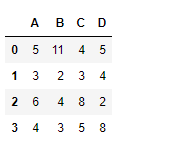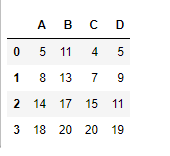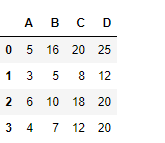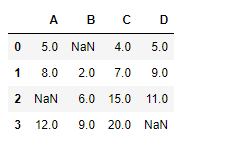Skip to content
Related Articles
Python | Pandas dataframe.cumsum()
• Last Updated : 16 Nov, 2018

Python is a great language for doing data analysis, primarily because of the fantastic ecosystem of data-centric python packages. Pandas is one of those packages and makes importing and analyzing data much easier.

Pandas` dataframe.cumsum()` is used to find the cumulative sum value over any axis. Each cell is populated with the cumulative sum of the values seen so far.

Syntax: DataFrame.cumsum(axis=None, skipna=True, *args, **kwargs)

Parameters:
axis : {index (0), columns (1)}
skipna : Exclude NA/null values. If an entire row/column is NA, the result will be NA

Returns: cumsum : Series

Example #1: Use `cumsum()` function to find the cumulative sum of the values along the index axis.

 `# importing pandas as pd``import` `pandas as pd`` ` `# Creating the dataframe``df ``=` `pd.DataFrame({``"A"``:[``5``, ``3``, ``6``, ``4``],``                   ``"B"``:[``11``, ``2``, ``4``, ``3``],``                   ``"C"``:[``4``, ``3``, ``8``, ``5``],``                   ``"D"``:[``5``, ``4``, ``2``, ``8``]})`` ` `# Print the dataframe``df`

Output :Now find the cumulative sum of the values over the index axis

 `# To find the cumulative sum``df.cumsum(axis ``=` `0``)`

Output :Example #2: Use `cumsum()` function to find the cumulative sum of the values seen so far along the column axis.

 `# importing pandas as pd``import` `pandas as pd`` ` `# Creating the dataframe``df ``=` `pd.DataFrame({``"A"``:[``5``, ``3``, ``6``, ``4``],``                   ``"B"``:[``11``, ``2``, ``4``, ``3``],``                   ``"C"``:[``4``, ``3``, ``8``, ``5``],``                   ``"D"``:[``5``, ``4``, ``2``, ``8``]})`` ` `# To find the cumulative sum along column axis``df.cumsum(axis ``=` `1``)`

Output :Example #3: Use `cumsum()` function to find the cumulative sum of the values seen so far along the index axis in a data frame with `NaN` value present in dataframe.

 `# importing pandas as pd``import` `pandas as pd`` ` `# Creating the dataframe``df ``=` `pd.DataFrame({``"A"``:[``5``, ``3``, ``None``, ``4``],``                   ``"B"``:[``None``, ``2``, ``4``, ``3``],``                   ``"C"``:[``4``, ``3``, ``8``, ``5``],``                   ``"D"``:[``5``, ``4``, ``2``, ``None``]})`` ` `# To find the cumulative sum``df.cumsum(axis ``=` `0``, skipna ``=` `True``)`

Output :The output is a dataframe with cells containing the cumulative sum of the values seen so far along the index axis. Any `Nan` value in the dataframe is skipped.

Attention geek! Strengthen your foundations with the Python Programming Foundation Course and learn the basics.

To begin with, your interview preparations Enhance your Data Structures concepts with the Python DS Course. And to begin with your Machine Learning Journey, join the Machine Learning – Basic Level Course

My Personal Notes arrow_drop_up